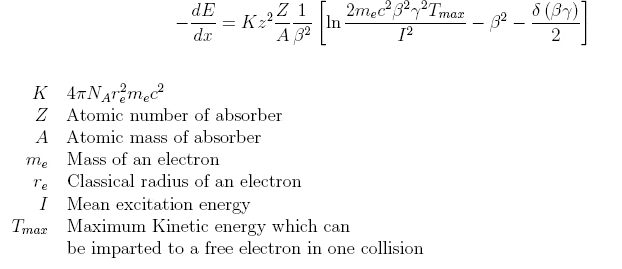# Range of a proton in matter

## Homework Statement

What thickness of copper is required to stop a 500 MeV/c proton?

E=(m^2+p^2)^1/2

## The Attempt at a Solution

So I have been to some websites and trying to google this- and everyone is using charts and looking of the value of the range in a material.

First, I cannot find the chart for copper and protons. I can convert the momentum to energy - but that's all I got.

How can I solve this problem? Thanks!

Did you find the Bethe(-Bloch) formula?Yes,,,, but how the heck am I suppose to use this formula??

There has to be got like a two line solution here for such a simple problem no?

Yes,,,, but how the heck am I suppose to use this formula??
Everything in there is constant, known, has some known approximations or depends on the energy of the particle only.
But the main point here: the energy loss does not depend so much on the material, it just depends on its density and it is proportional to density. That allows to compare the stopping distance of different materials.

The length of the solution depends on the approach and how much you can look up somewhere.

Hmm... So what is the variable I am trying to solve for then ? I want the thickness of copper so that has an Atomic mass: 63.546, and 29 Protons - So i have my A and Z.

and Tmax, I and the delta function.. What am I suppose to do with this. And even if I do plug everything in then I get -dE/dx...The derivative of E with respect to x. Is that what I want ?!?

Hmm... So what is the variable I am trying to solve for then ?
Well, ultimatively, the distance until the protons stop.
And even if I do plug everything in then I get -dE/dx...The derivative of E with respect to x. Is that what I want ?!?
If you know the energy loss per distance as function of energy, you can follow your proton through the material and evaluate its energy everywhere. It stops at the point where the energy reaches zero.

But I don't see an x in that equation, and I have no idea what beta, Tmax, I or how to use the delta function for that is. So I don't have all the values... very very confused, can you help me with step one? Just setting up the equation.

But I don't see an x in that equation
Left side, in the denominator.
If dE/dx would be a constant 10 MeV/cm, then your protons would have a range of 50cm, for example.

For the other values, there are typical values you can use. That won't be exact, but a good approximation.

Maybe this article will help clear up some confusion on the part of the OP:

http://www.kip.uni-heidelberg.de/~coulon/Lectures/Detectors/Free_PDFs/Lecture2.pdf [Broken]

Last edited by a moderator:
•mfb
Ya, see - I don't want to be rude but you are skipping some key details for me- like the part I am missing is how you are going from dE/dx = 10 MeV/cm to a range of 50cm. You say all that stuff are just constants specific to my problem. Then I wouldn't I have -dE/dx = CONSTANT. So E=Constant*x ? I am missing a lot of connection here. I read the article SteamKing supplied but again there is no examples or anything. Just a lot of data and charts which is hard to put into context of this particular problem. I am not stupid, I just need to see a worked out example and then it will make so much more sense to me I am sure. Like going back to my problem- I am still not sure how to even start that! Can we do the first few steps so I have somewhere to go from... THANKS

like the part I am missing is how you are going from dE/dx = 10 MeV/cm to a range of 50cm.
If you have 500 MeV, and lose 10 MeV of this energy every centimeter, then the particle can travel for 50 centimeters.
Then I wouldn't I have -dE/dx = CONSTANT
You don't have that, I made a simplified example that is not directly applicable to your problem.

Ignore the details for now, focus on the main part: the energy loss does not depend much on microscopic details of the material, it mainly depends on the density. So 1cm in a material of density 10g/cm^3 will give the same energy loss as 10 cm in a material of density 1g/cm^3 (arbitrary values here), and all other distances scale in the same way, therefore the stopping distance will be 10 times longer in the thinner material as well.
If you can find tables for the stopping distance of 500 MeV protons in one arbitrary material, you can scale this to copper. Otherwise you'll have to look at the energy-dependence of the energy loss again.